Data visualization has never been more widespread and consumed by a global audience as it has been this year with the Coronavirus pandemic. If you are interested in the statistics behind many of the numbers you see displayed in data visualizations then please reference my colleague’s blog series:

One visualization that is commonly used to display metrics of Coronavirus is a bar line chart where the bars display the actual values and the line is a moving average metric.

The screenshot below shows the number of web visits per week and a 5 week moving average value. I’ve named it Visits (5 Week Moving Avg Bracket) since I am including both past and present data points into this calculation.

I will demonstrate how to easily generate this moving average metric to use in your SAS Visual Analytics reports.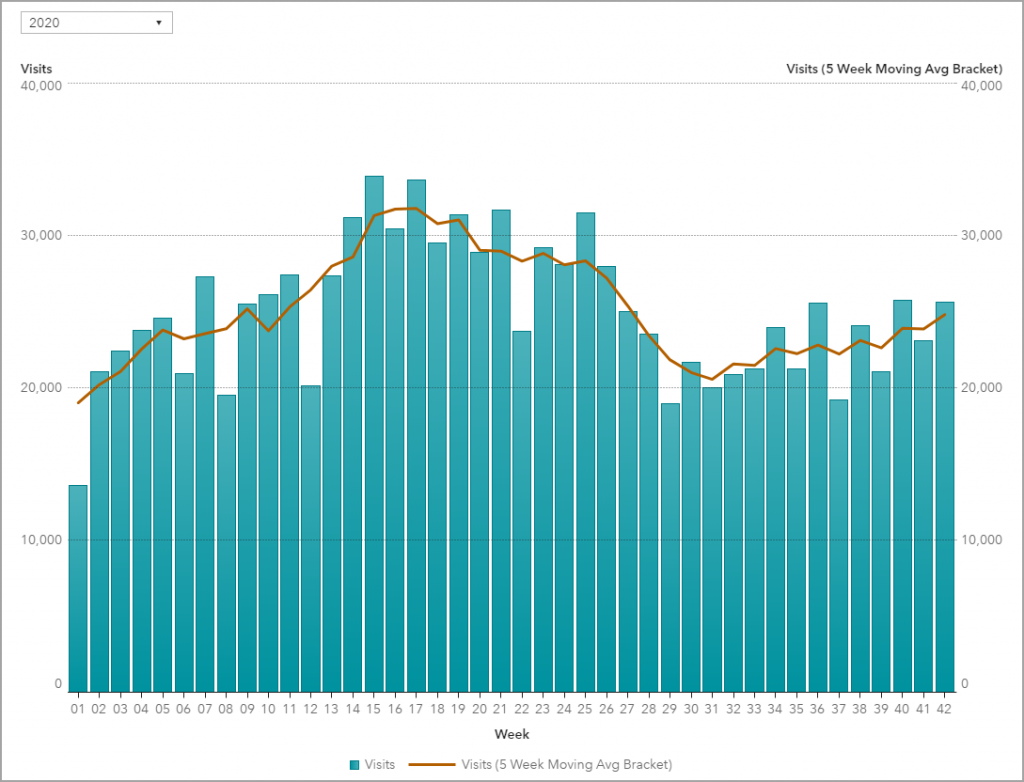## Moving Average

Moving average is essentially a block of data points averaged together, creating a series of averages for the data. This is commonly used with time series data to smooth out short-term fluctuations and highlight longer-term trends or cycles.

Traditionally, the moving average block takes the current data point and then moves forward in the series, which is often the case in financial applications. However, it is more common in science and engineering to take equal data points before and after the current position, creating what I am calling a moving average bracket.

SAS’ Visual Analytics calculation gives you the flexibility to define how to average the data points for your moving average: how many positions prior and/or after the current data point.

## Steps to generate the Moving Average

From the Data pane, right-click on the measure you want to generate the moving average and select New calculation.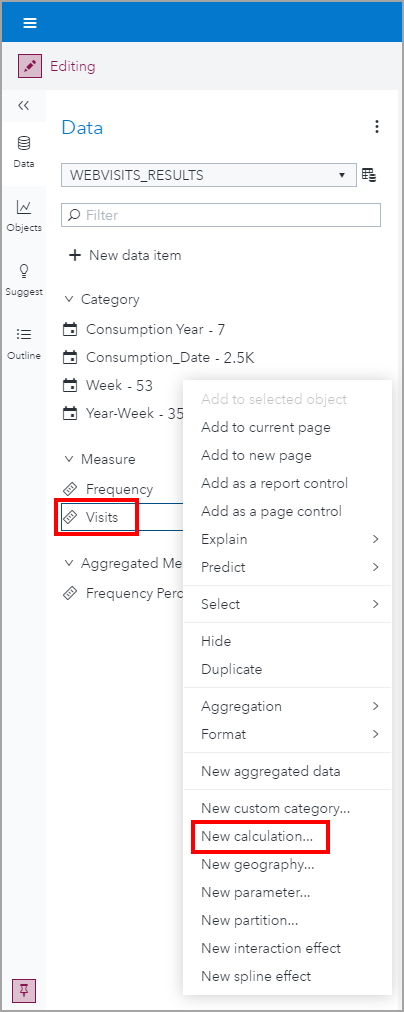Next, in the Create Calculation window:

• Name: Visits (5 Week Moving Avg Bracket) (a meaningful name of your choice)
• Type: Moving average
• Number of cells to average: 5 (or the number of your choice)

Click OK.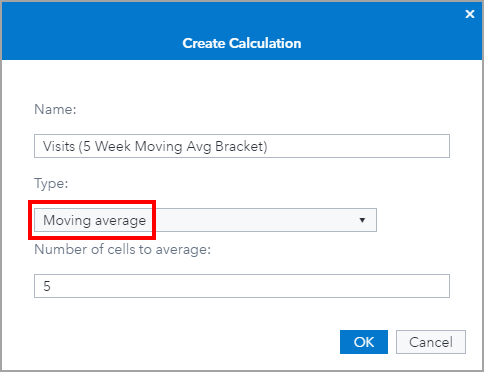Notice that you did not have the option to specify how many data points to include before and after the current position. To do that, we will right-click on the generated calculation and select Edit.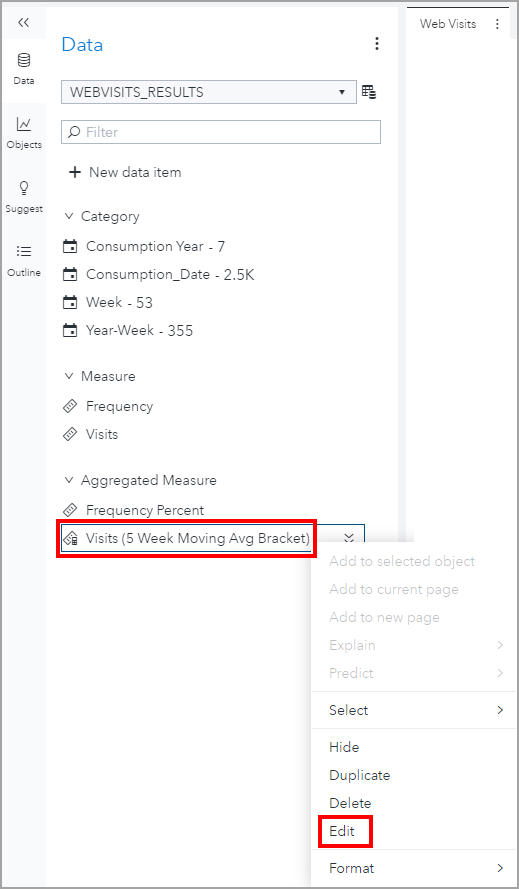From the Edit Calculated Item window, make your starting and ending point position selections. The current position is denoted as zero. In my example, I want a 5 point moving average bracket, therefore I want to average the two positions before current, (-2), current, and then the two positions after current (2).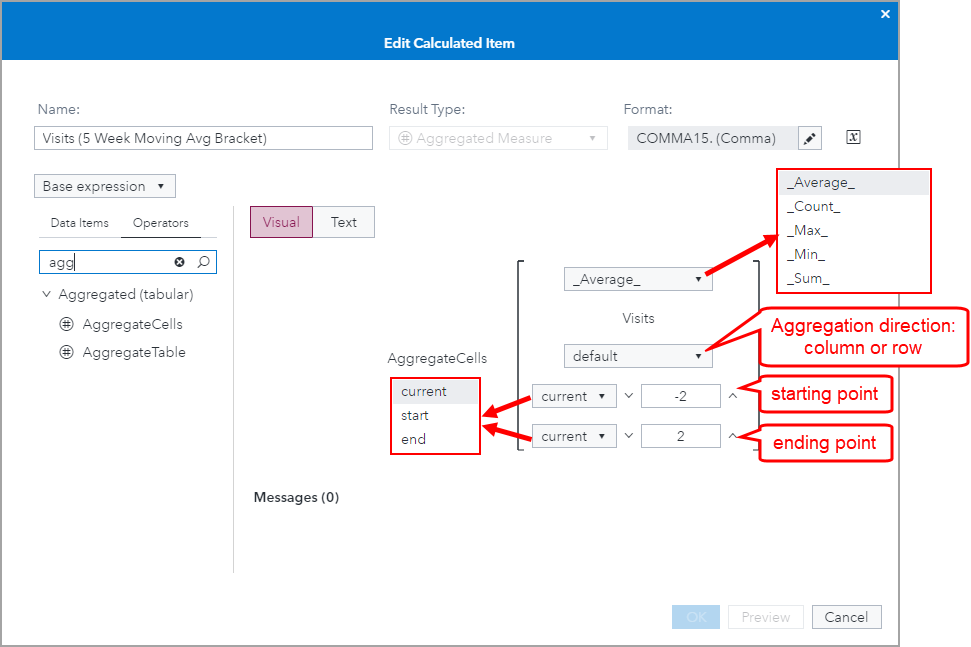Success. We now have generated our 5 point moving average bracket. You may be wondering, where do you specify the time frame? Granted I named this metric Visits (5 Week Moving Average Bracket) but I did not specify the week time metric anywhere. This is because, for this aggregated measure, the time duration is directly dependent on the data items you have assigned to the object.

In my example, for my web visit data, I want to look at the aggregation at the week level, therefore this Aggregate Cell measure will be grouped by week. I wanted to name my measure appropriately so that any user reading the visual knows the time frame that is being averaged.

If you are unsure of how you want to aggregate your time series, or you are allowing your report viewers the ability to change the role assignments through self-service reporting (see blog or YouTube for more information) then it would be best if you name your measure as 5 Point Moving Average Bracket and leave off the aggregation of the time series.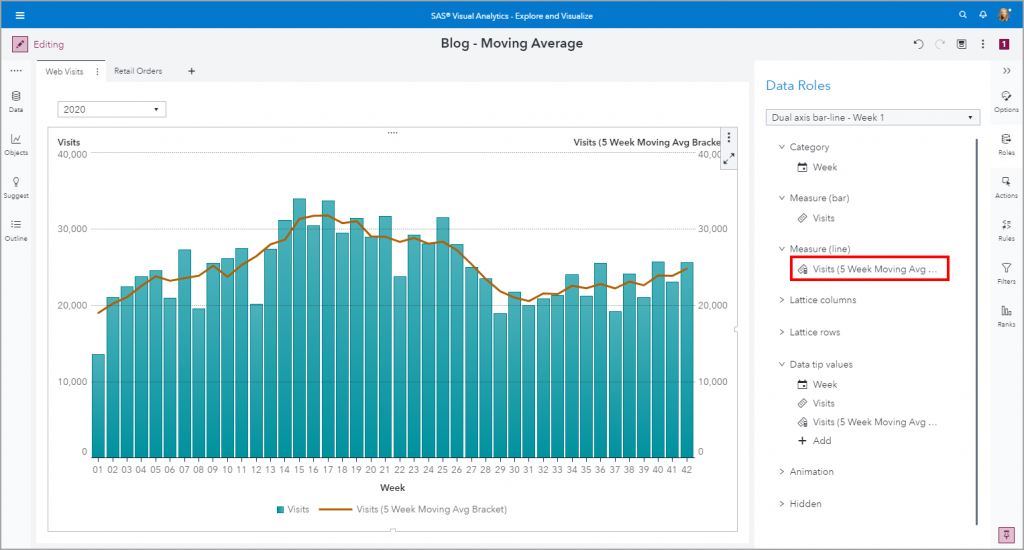Let’s take a look at what the numbers are behind the scene. I’ve expanded the object to its Maximize mode so that I can see the summary table of the metrics that make up this object.

The data points will average until the full bracket size is met, then it will slide that bracket down the time series. Keep in mind that our bracket size is five points, two previous data points (-2), current data point (0), and two future data points (2).

Let’s look at week 01. Week 01 is our current, or zero, position. We do not have any prior data points so the only points that can be used from the bracket are positions 1 and 2. See the first highlighted yellow Visits (5 Week Moving Avg Bracket) and how it corresponds to the Visits.

Next, week 02 is able to include a -1 position into the bracket. The full moving average bracket isn’t met till week 03 where all of the data points: -2 through 2 are available. Then the moving average bracket slides along the rest of the time series.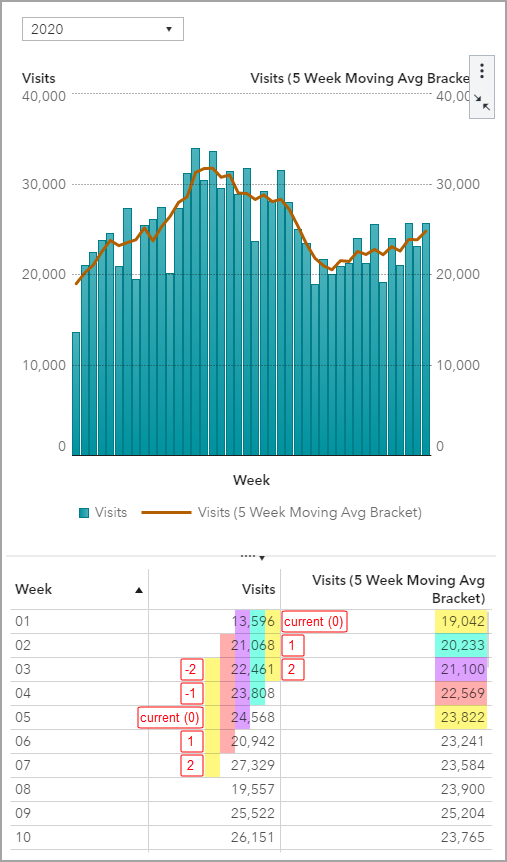Seeing the object’s summary data and the breakdown of how the moving average bracket is calculated for the data should drive home the fact that the time period aggregation is solely driven by the object’s role assignments.

In my second example, I am looking at retail data and this is aggregated to the day level.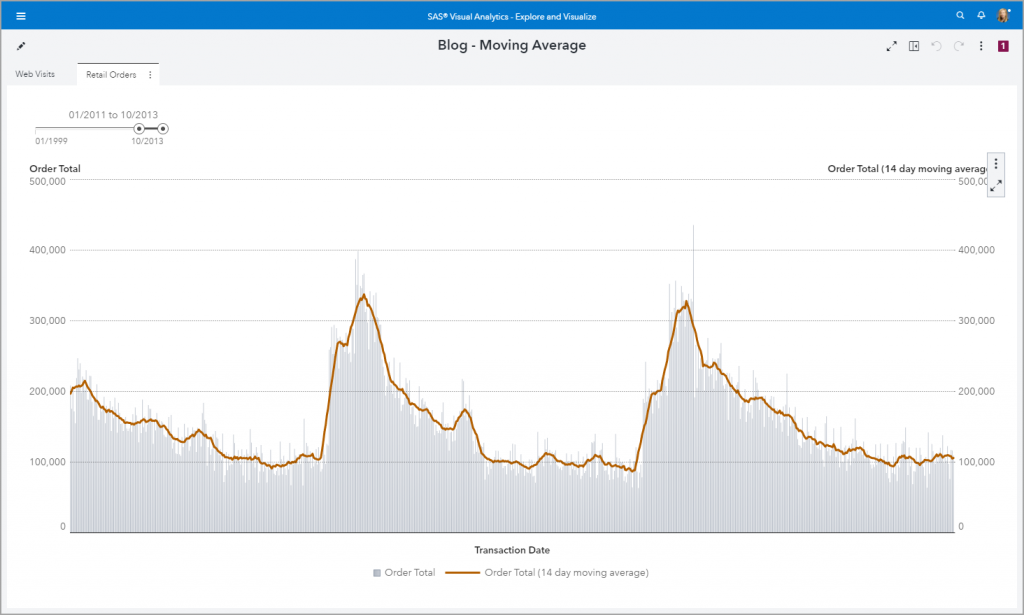I chose to demonstrate a 14 day moving average bracket.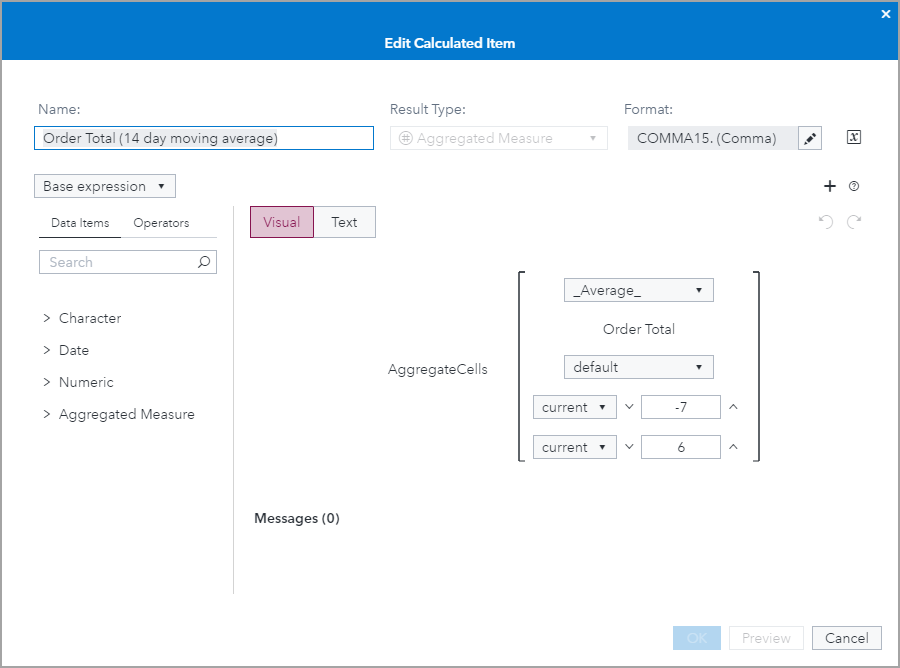## Bar Line Chart

In both of my examples, I used the Dual axis bar-line chart object. Default Y axis behavior for bar charts is to start at zero, but line charts since they are intended to show trend can start at a value other than zero. Use the Options pane to select your desired line chart baseline. I choose to have both my bar and line charts fixed at zero. Explore the other Options to further customize your visual.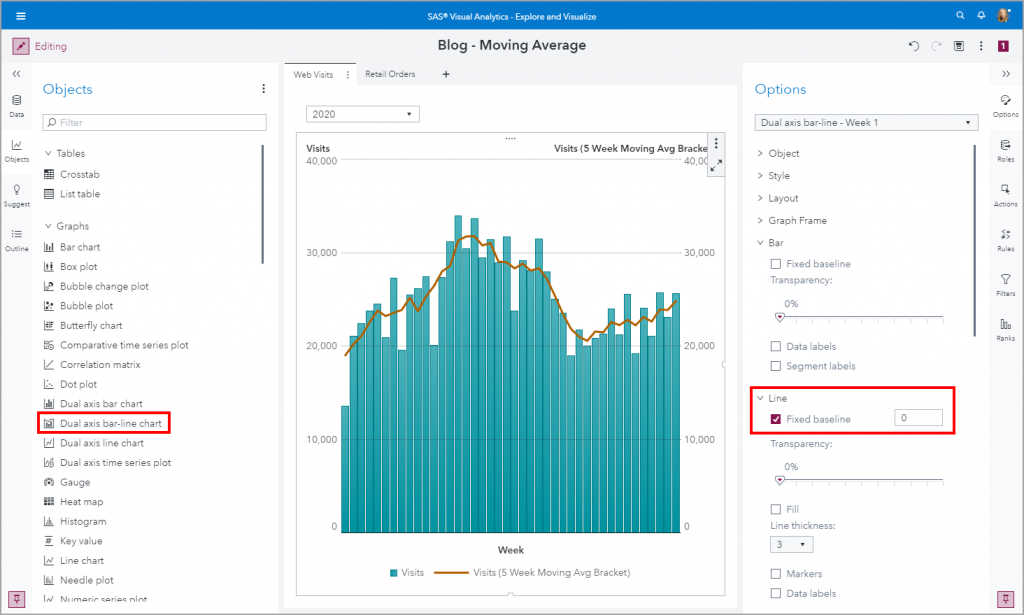## Summary

Calculating the moving average is offered as a SAS Visual Analytics out-of-the-box Derived Item. See the SAS Documentation for more information about Derived Items.

Once you’ve specified the window of data points to average for the moving average you can go back and edit the starting and ending points around the current position. Remember that you will not be specifying a time frame for the aggregation but it will be dynamically determined based on the role assignments to the visual.

If you are interested in other uses for the moving average operator Aggregate Cells take a look at this article: VA Report Example: Moving 30 Day Rolling Sum.

Also, check out these resources:

SAS Visual Analytics example: moving average was published on SAS Users.With time series data analysis, we can apply moving average methods to predict data points without seasonality. This includes Simple Average (SA), Simple Moving Average (SMA), Weighted Moving Average (WMA), Exponential Moving Average (EMA), etc. For series with a trend but without seasonality, we can use linear, non-linear and autoregressive prediction models. In practice, we often use these kinds of moving average methods for series data with large variance, e.g. financial and stock market data.

Since introducing the window concept, SMA reflects historical data with a lag problem, and all points in the same window have the same importance to the current point. Weights are introduced to reflect the different importances of points in a window, and it leads to WMA and EMA. WMA gives greater weight to recent observations while less weight to the longer-term observations. The weights decrease in a linear fashion. If the sequence fluctuations are not large, the same weight is given to the observation and WMA degenerates into a SMA and the WMA becomes an EMA when weights decrease exponentially. However, these traditional moving average methods ave a lag problem due to introducing the smooth window concept and its results are less responsive to the current value. To gain a better balance between lag reduction and curve smoothing in a moving average, we must get help from some smarter mathematics algorithm.

## What is Hull Moving Average?

Hull Moving Average (HMA) is a fast moving average method with low lag developed by Australia investor Alan Hull in 2005. It’s known to almost eliminate lag altogether and manages to improve smoothing at the same time. Longer period HMAs can be used as an indicator to identify trend, and shorter period HMAs can be used as entry signals in the direction of the prevailing trend. Due to lag problems in all moving average methods, it’s not suggested to be used as a reverse signal alone. Anyway, we can use different window HMAs to build a more robust trading strategy. E.g., 52-week HMA for trend indicator, and 13-week HMA for entry signal. Here is how it works:

• Long period HMA identifies the trend: rising HMA indicates the prevailing trend is rising, it’s better to enter long positions; falling HMA indicate the prevailing trend is falling, it’s better to enter short positions.
• Short period HMA identifies the entry signals in the direction of the prevailing trend: When the prevailing trend is rising, HMA goes up to indicate a long entry signal. When the prevailing trend is falling, HMA goes down to indicate a short entry signal.

Both long & short period HMAs in bullish mode generate Long Signal, and both in bearish mode generate Short Signal. So Long Trades get a buy signal after a Long Signal occurs, while Short Trades get a sell signal after a Short Signal occurs. Long Trades get a Sell Signal when Long or Short Trend is no longer bullish and Short Trades get a buy signal when Long or Short trend is no longer bearish. Figure 1 is a sample for this HMA based trading strategy.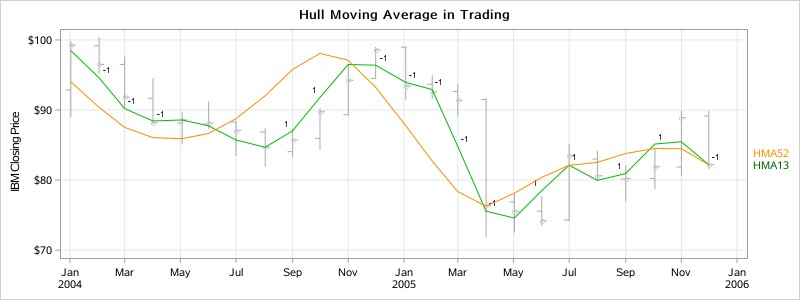In fact, HMA is quite simple. Its calculation only includes three WMA steps:

1. Calculate WMA of original sequence X using window length n ;
2. Calculate WMA of X using window length n/2;
3. Calculate WMA of derived sequence Y using window length Sqrt(n), while Y is the new series of 2 * WMA(x, n/2) – WMA(x, n). So HMA(x, n) formula can be defined as below:

HMA(x, n) = WMA( 2*WMA(x, n/2) − WMA(x, n), sqrt(n) )

HMA makes the moving average line more responsive to current value and keeps the smoothing of the curve line. It almost eliminates lag altogether and manages to improve smoothing at the same time.

## How does HMA achieve the perfect balance?

We know SMA can gain the curve line of an average value for historical data falling in the current window, but it has the constant lag of at least a half window. The output may also have poor smoothness. If we embed SMA multiple times to get the average of the averages, it would be smoother but would increase lag at the same time.

Suppose we have this series: {1, 2, 3, 4, 5, 6, 7, 8, 9, 10}. The SMA at the last point is 5.5 with window length n=10, i.e. SUM[1...10]/10
is much different than the real value 10. If we reduce the window length to n=5, the SMA at the last point is 8, i.e. SUM[6…10]/5. If we have the second SMA with half size window and add the difference between these two SMAs, then we can get 8 + (8-5.5)=10.5, which is very close to the real value 10 and will eliminate lag. Then we can use SMA with specific window length again to reduce that slight overcompensation and improve smoothness. HMA uses linear WMA instead of SMA, and the last window length is sqrt(n). This is the key trick with using HMA.

Figure 2 below shows the difference between HMA(x, 4) and embed SMA twice SMA( SMA(x,4),4).

In stock trading, there are lots of complicated technical indicators derived from stock historical data, such as Ease of Movement indicators (EMV), momentum indicators (MTM), MACD indicators, Energy indicator CR, KDJ indicators, Bollinger (BOL) and so on. Its essence is like feature engineering extraction before neural network training. The fundamental purpose is to find some intrinsic property from price fluctuation. The Hull moving average itself was discovered and applied to real trading practices, which gives us a glimpse to the complication of building an automatic financial trading strategy under the veil. It’s just the beginning, the reflex in trading and aging of models may both bring uncertainty, so the effectiveness of quantitative trading strategies is a very complex topic which needs to be validated through real practice.

## HMA in SAS Code

SAS was created for data analysis however, and my colleagues go into detail about how data analysis connects with HMA. Check out Cindy Wang's How to Calculate Hull Moving Average in SAS Visual Analytics and Rick Wicklin's The Hull moving average: Implement a custom time series smoother in SAS. I would like to share how to implement both HMA/WMA with only 25 lines of code in this article. You can use the macro anywhere to build HMA series with specific window lengths.

First, implement Weighted Moving Average (WMA) with SAS macro as shown below (only 11 lines). The macro has four arguments:

• WMA_N       WMA window length
• INPUT          Input variable name
• OUT             Output variable name, default is input name with _WMA_n suffix
• OUTLABEL The label of output variable, default is “input name WMA(n)
```%macro AddWMA(WMA_N=4, INPUT=close, OUT=&INPUT._WMA_&WMA_N., OUTLABEL=&INPUT. WMA(&WMA_N.)); retain _sumx_&INPUT._WMA_&WMA_N.; _sumx_&INPUT._WMA_&WMA_N.=sum (_sumx_&INPUT._WMA_&WMA_N., &INPUT., -lag&WMA_N.( &INPUT. )) ;   retain _sum_&INPUT._WMA_&WMA_N.; _sum_&INPUT._WMA_&WMA_N.=sum (_sum_&INPUT._WMA_&WMA_N., &WMA_N * &INPUT., -lag( _sumx_&INPUT._WMA_&WMA_N. )) ;   retain _sumw_&INPUT._WMA_&WMA_N.; if _N_ <= &WMA_N then _sumw_&INPUT._WMA_&WMA_N. = sum (_sumw_&INPUT._WMA_&WMA_N., &WMA_N, -_N_, 1);   &OUT=_sum_&INPUT._WMA_&WMA_N. / _sumw_&INPUT._WMA_&WMA_N.;   drop _sumx_&INPUT._WMA_&WMA_N. _sumw_&INPUT._WMA_&WMA_N. _sum_&INPUT._WMA_&WMA_N.; label &OUT="&OUTLABEL"; %mend;```

We must watch out for the trick in the upper implementation of WMA with linear weights. In fact, there is no do-loop in the code, and it also doesn’t perform repeat computations for weights and values. We just keep the last sum of all values in the window (See Ln2-3), and the sum for WMA is the last sum for WMA plus window length times the value subtracted from it (See Ln4-5). For weight sum computation, we just need to pay attention to the points less than the window length(See Ln6-7).

Second, implement HMA with three times WMA invocation as below, (only 9 lines). The macro has four arguments:

• HMA_N       HMA window length
• INPUT         Input variable name
• OUT            Output variable name, default is input name with _HMA_n suffix
• OUTLABEL The label of output variable, default is “input name HMA(n)
```%macro AddHMA(HMA_N=5, INPUT=close, OUT=&INPUT._HMA_&HMA_N., outlabel=&INPUT. HMA(&HMA_N.)); %AddWMA(WMA_N=&HMA_N, INPUT=&INPUT.);   %AddWMA(WMA_N=%sysfunc(round(&HMA_N./2)), INPUT=&INPUT.);   &INPUT._HMA_&HMA_N._DELTA=2 * &INPUT._WMA_%sysfunc(round(&HMA_N./2)) - &INPUT._WMA_&HMA_N; %AddWMA(WMA_N=%sysfunc(round(%sysfunc(sqrt(&HMA_N)))), INPUT=&INPUT._HMA_&HMA_N._DELTA);   rename &INPUT._HMA_&HMA_N._DELTA_WMA_%sysfunc(round(%sysfunc(sqrt(&HMA_N.))))=&OUT; label &INPUT._HMA_&HMA_N._DELTA_WMA_%sysfunc(round(%sysfunc(sqrt(&HMA_N.))))="&outlabel";   drop &INPUT._WMA_&HMA_N &INPUT._WMA_%sysfunc(round(&HMA_N./2)) &INPUT._HMA_&HMA_N._DELTA; %mend;```

The upper code is very intuitive as the first two lines perform WMA with window n and n/2 for X, then the next two lines generate new series Y and performs WMA with window sqrt(n). Other codes are just to improve macro flexibility and to drop temp variables, etc.

To compare HMA with SMA result, we must also implement SMA with the 7 lines of code as shown below. It has the same argument as WMA/HMA above, but SMA is an unnecessary part of HMA macro %ADDHMA implmentation.

```  %macro AddMA(MA_N=5, INPUT=close, out=&INPUT._MA_&MA_N., outlabel=&INPUT. MA(&MA_N.)); retain _sum_&INPUT._MA_&MA_N.; _sum_&INPUT._MA_&MA_N.=sum (_sum_&INPUT._MA_&MA_N., &INPUT., -lag&MA_N.( &INPUT. )) ; &out=_sum_&INPUT._MA_&MA_N. / min(_n_, &MA_N.);   drop _sum_&INPUT._MA_&MA_N.; label &out="&outlabel"; %mend;```

Now we can test all the above implementations as shown below. First is a simple case we described above:

```data a; input x @@; %AddMA( input=x, MA_N=5, out=SMA, outlabel=%str(SMA)); %AddHMA( input=x, HMA_N=5, out=HMA, outlabel=%str(HMA)) datalines; 1 2 3 4 5 6 7 8 9 10 ; proc print data=a label; format _all_ 8.2; run;```

The output is listed below, the HMA result is much more close to the real value x than SMA. It’s more responsive and less in lag.

Now we can use the HMA macro to check IBM stock data in the SAS system dataset SASHELP.Stocks and draw trends in the plot to compare HMA(x, 5) and SMA(x, 5) output.

```data Stocks_HMA; set sashelp.Stocks(where=(stock='IBM')); %AddHMA( HMA_N=5, out=HMA, outlabel=%str(HMA)) %AddMA(MA_N=5, out=SMA, outlabel=%str(SMA)); run; ods graphics / width=800px height=300px; title "Comparison of Simple and Hull Moving Average"; proc sgplot data=Stocks_HMA noautolegend; highlow x=Date high=High low=Low / lineattrs=(color=LightGray); scatter x=Date y=Close / markerattrs=(color=LightGray); series x=Date y=SMA / curvelabel curvelabelattrs=(color=DarkGreen) lineattrs=(color=DarkGreen); series x=Date y=HMA / curvelabel curvelabelattrs=(color=DarkOrange) lineattrs=(color=DarkOrange); refline '01JUN1992'd / axis=x label='June 1992' labelloc=inside; xaxis grid display=(nolabel); yaxis grid max=200 label="IBM Closing Price"; run;```

## Summary

We have talked about what Hull Moving Average is, how it works, and worked through a super simple SAS Macro implementation for HMA, WMA and SMA with only 25 lines of SAS code. This HMA implementation makes it quite simple and efficient to calculate HMA in SAS Data step without SAS/IML and Visual Analytics. In fact, it shows how easy process rolling-window computation for time series data can be in SAS, and opens a new window to build complicated technical indicators for stock trading systems yourself.

Implementing HMA, WMA & SMA with 25 lines of SAS code was published on SAS Users.With time series data analysis, we can apply moving average methods to predict data points without seasonality. This includes Simple Average (SA), Simple Moving Average (SMA), Weighted Moving Average (WMA), Exponential Moving Average (EMA), etc. For series with a trend but without seasonality, we can use linear, non-linear and autoregressive prediction models. In practice, we often use these kinds of moving average methods for series data with large variance, e.g. financial and stock market data.

Since introducing the window concept, SMA reflects historical data with a lag problem, and all points in the same window have the same importance to the current point. Weights are introduced to reflect the different importances of points in a window, and it leads to WMA and EMA. WMA gives greater weight to recent observations while less weight to the longer-term observations. The weights decrease in a linear fashion. If the sequence fluctuations are not large, the same weight is given to the observation and WMA degenerates into a SMA and the WMA becomes an EMA when weights decrease exponentially. However, these traditional moving average methods ave a lag problem due to introducing the smooth window concept and its results are less responsive to the current value. To gain a better balance between lag reduction and curve smoothing in a moving average, we must get help from some smarter mathematics algorithm.

## What is Hull Moving Average?

Hull Moving Average (HMA) is a fast moving average method with low lag developed by Australia investor Alan Hull in 2005. It’s known to almost eliminate lag altogether and manages to improve smoothing at the same time. Longer period HMAs can be used as an indicator to identify trend, and shorter period HMAs can be used as entry signals in the direction of the prevailing trend. Due to lag problems in all moving average methods, it’s not suggested to be used as a reverse signal alone. Anyway, we can use different window HMAs to build a more robust trading strategy. E.g., 52-week HMA for trend indicator, and 13-week HMA for entry signal. Here is how it works:

• Long period HMA identifies the trend: rising HMA indicates the prevailing trend is rising, it’s better to enter long positions; falling HMA indicate the prevailing trend is falling, it’s better to enter short positions.
• Short period HMA identifies the entry signals in the direction of the prevailing trend: When the prevailing trend is rising, HMA goes up to indicate a long entry signal. When the prevailing trend is falling, HMA goes down to indicate a short entry signal.

Both long & short period HMAs in bullish mode generate Long Signal, and both in bearish mode generate Short Signal. So Long Trades get a buy signal after a Long Signal occurs, while Short Trades get a sell signal after a Short Signal occurs. Long Trades get a Sell Signal when Long or Short Trend is no longer bullish and Short Trades get a buy signal when Long or Short trend is no longer bearish. Figure 1 is a sample for this HMA based trading strategy.In fact, HMA is quite simple. Its calculation only includes three WMA steps:

1. Calculate WMA of original sequence X using window length n ;
2. Calculate WMA of X using window length n/2;
3. Calculate WMA of derived sequence Y using window length Sqrt(n), while Y is the new series of 2 * WMA(x, n/2) – WMA(x, n). So HMA(x, n) formula can be defined as below:

HMA(x, n) = WMA( 2*WMA(x, n/2) − WMA(x, n), sqrt(n) )

HMA makes the moving average line more responsive to current value and keeps the smoothing of the curve line. It almost eliminates lag altogether and manages to improve smoothing at the same time.

## How does HMA achieve the perfect balance?

We know SMA can gain the curve line of an average value for historical data falling in the current window, but it has the constant lag of at least a half window. The output may also have poor smoothness. If we embed SMA multiple times to get the average of the averages, it would be smoother but would increase lag at the same time.

Suppose we have this series: {1, 2, 3, 4, 5, 6, 7, 8, 9, 10}. The SMA at the last point is 5.5 with window length n=10, i.e. SUM[1...10]/10
is much different than the real value 10. If we reduce the window length to n=5, the SMA at the last point is 8, i.e. SUM[6…10]/5. If we have the second SMA with half size window and add the difference between these two SMAs, then we can get 8 + (8-5.5)=10.5, which is very close to the real value 10 and will eliminate lag. Then we can use SMA with specific window length again to reduce that slight overcompensation and improve smoothness. HMA uses linear WMA instead of SMA, and the last window length is sqrt(n). This is the key trick with using HMA.

Figure 2 below shows the difference between HMA(x, 4) and embed SMA twice SMA( SMA(x,4),4).

In stock trading, there are lots of complicated technical indicators derived from stock historical data, such as Ease of Movement indicators (EMV), momentum indicators (MTM), MACD indicators, Energy indicator CR, KDJ indicators, Bollinger (BOL) and so on. Its essence is like feature engineering extraction before neural network training. The fundamental purpose is to find some intrinsic property from price fluctuation. The Hull moving average itself was discovered and applied to real trading practices, which gives us a glimpse to the complication of building an automatic financial trading strategy under the veil. It’s just the beginning, the reflex in trading and aging of models may both bring uncertainty, so the effectiveness of quantitative trading strategies is a very complex topic which needs to be validated through real practice.

## HMA in SAS Code

SAS was created for data analysis however, and my colleagues go into detail about how data analysis connects with HMA. Check out Cindy Wang's How to Calculate Hull Moving Average in SAS Visual Analytics and Rick Wicklin's The Hull moving average: Implement a custom time series smoother in SAS. I would like to share how to implement both HMA/WMA with only 25 lines of code in this article. You can use the macro anywhere to build HMA series with specific window lengths.

First, implement Weighted Moving Average (WMA) with SAS macro as shown below (only 11 lines). The macro has four arguments:

• WMA_N       WMA window length
• INPUT          Input variable name
• OUT             Output variable name, default is input name with _WMA_n suffix
• OUTLABEL The label of output variable, default is “input name WMA(n)
```%macro AddWMA(WMA_N=4, INPUT=close, OUT=&INPUT._WMA_&WMA_N., OUTLABEL=&INPUT. WMA(&WMA_N.)); retain _sumx_&INPUT._WMA_&WMA_N.; _sumx_&INPUT._WMA_&WMA_N.=sum (_sumx_&INPUT._WMA_&WMA_N., &INPUT., -lag&WMA_N.( &INPUT. )) ;   retain _sum_&INPUT._WMA_&WMA_N.; _sum_&INPUT._WMA_&WMA_N.=sum (_sum_&INPUT._WMA_&WMA_N., &WMA_N * &INPUT., -lag( _sumx_&INPUT._WMA_&WMA_N. )) ;   retain _sumw_&INPUT._WMA_&WMA_N.; if _N_ <= &WMA_N then _sumw_&INPUT._WMA_&WMA_N. = sum (_sumw_&INPUT._WMA_&WMA_N., &WMA_N, -_N_, 1);   &OUT=_sum_&INPUT._WMA_&WMA_N. / _sumw_&INPUT._WMA_&WMA_N.;   drop _sumx_&INPUT._WMA_&WMA_N. _sumw_&INPUT._WMA_&WMA_N. _sum_&INPUT._WMA_&WMA_N.; label &OUT="&OUTLABEL"; %mend;```

We must watch out for the trick in the upper implementation of WMA with linear weights. In fact, there is no do-loop in the code, and it also doesn’t perform repeat computations for weights and values. We just keep the last sum of all values in the window (See Ln2-3), and the sum for WMA is the last sum for WMA plus window length times the value subtracted from it (See Ln4-5). For weight sum computation, we just need to pay attention to the points less than the window length(See Ln6-7).

Second, implement HMA with three times WMA invocation as below, (only 9 lines). The macro has four arguments:

• HMA_N       HMA window length
• INPUT         Input variable name
• OUT            Output variable name, default is input name with _HMA_n suffix
• OUTLABEL The label of output variable, default is “input name HMA(n)
```%macro AddHMA(HMA_N=5, INPUT=close, OUT=&INPUT._HMA_&HMA_N., outlabel=&INPUT. HMA(&HMA_N.)); %AddWMA(WMA_N=&HMA_N, INPUT=&INPUT.);   %AddWMA(WMA_N=%sysfunc(round(&HMA_N./2)), INPUT=&INPUT.);   &INPUT._HMA_&HMA_N._DELTA=2 * &INPUT._WMA_%sysfunc(round(&HMA_N./2)) - &INPUT._WMA_&HMA_N; %AddWMA(WMA_N=%sysfunc(round(%sysfunc(sqrt(&HMA_N)))), INPUT=&INPUT._HMA_&HMA_N._DELTA);   rename &INPUT._HMA_&HMA_N._DELTA_WMA_%sysfunc(round(%sysfunc(sqrt(&HMA_N.))))=&OUT; label &INPUT._HMA_&HMA_N._DELTA_WMA_%sysfunc(round(%sysfunc(sqrt(&HMA_N.))))="&outlabel";   drop &INPUT._WMA_&HMA_N &INPUT._WMA_%sysfunc(round(&HMA_N./2)) &INPUT._HMA_&HMA_N._DELTA; %mend;```

The upper code is very intuitive as the first two lines perform WMA with window n and n/2 for X, then the next two lines generate new series Y and performs WMA with window sqrt(n). Other codes are just to improve macro flexibility and to drop temp variables, etc.

To compare HMA with SMA result, we must also implement SMA with the 7 lines of code as shown below. It has the same argument as WMA/HMA above, but SMA is an unnecessary part of HMA macro %ADDHMA implmentation.

```  %macro AddMA(MA_N=5, INPUT=close, out=&INPUT._MA_&MA_N., outlabel=&INPUT. MA(&MA_N.)); retain _sum_&INPUT._MA_&MA_N.; _sum_&INPUT._MA_&MA_N.=sum (_sum_&INPUT._MA_&MA_N., &INPUT., -lag&MA_N.( &INPUT. )) ; &out=_sum_&INPUT._MA_&MA_N. / min(_n_, &MA_N.);   drop _sum_&INPUT._MA_&MA_N.; label &out="&outlabel"; %mend;```

Now we can test all the above implementations as shown below. First is a simple case we described above:

```data a; input x @@; %AddMA( input=x, MA_N=5, out=SMA, outlabel=%str(SMA)); %AddHMA( input=x, HMA_N=5, out=HMA, outlabel=%str(HMA)) datalines; 1 2 3 4 5 6 7 8 9 10 ; proc print data=a label; format _all_ 8.2; run;```

The output is listed below, the HMA result is much more close to the real value x than SMA. It’s more responsive and less in lag.

Now we can use the HMA macro to check IBM stock data in the SAS system dataset SASHELP.Stocks and draw trends in the plot to compare HMA(x, 5) and SMA(x, 5) output.

```data Stocks_HMA; set sashelp.Stocks(where=(stock='IBM')); %AddHMA( HMA_N=5, out=HMA, outlabel=%str(HMA)) %AddMA(MA_N=5, out=SMA, outlabel=%str(SMA)); run; ods graphics / width=800px height=300px; title "Comparison of Simple and Hull Moving Average"; proc sgplot data=Stocks_HMA noautolegend; highlow x=Date high=High low=Low / lineattrs=(color=LightGray); scatter x=Date y=Close / markerattrs=(color=LightGray); series x=Date y=SMA / curvelabel curvelabelattrs=(color=DarkGreen) lineattrs=(color=DarkGreen); series x=Date y=HMA / curvelabel curvelabelattrs=(color=DarkOrange) lineattrs=(color=DarkOrange); refline '01JUN1992'd / axis=x label='June 1992' labelloc=inside; xaxis grid display=(nolabel); yaxis grid max=200 label="IBM Closing Price"; run;```

## Summary

We have talked about what Hull Moving Average is, how it works, and worked through a super simple SAS Macro implementation for HMA, WMA and SMA with only 25 lines of SAS code. This HMA implementation makes it quite simple and efficient to calculate HMA in SAS Data step without SAS/IML and Visual Analytics. In fact, it shows how easy process rolling-window computation for time series data can be in SAS, and opens a new window to build complicated technical indicators for stock trading systems yourself.

Implementing HMA, WMA & SMA with 25 lines of SAS code was published on SAS Users.With time series data analysis, we can apply moving average methods to predict data points without seasonality. This includes Simple Average (SA), Simple Moving Average (SMA), Weighted Moving Average (WMA), Exponential Moving Average (EMA), etc. For series with a trend but without seasonality, we can use linear, non-linear and autoregressive prediction models. In practice, we often use these kinds of moving average methods for series data with large variance, e.g. financial and stock market data.

Since introducing the window concept, SMA reflects historical data with a lag problem, and all points in the same window have the same importance to the current point. Weights are introduced to reflect the different importances of points in a window, and it leads to WMA and EMA. WMA gives greater weight to recent observations while less weight to the longer-term observations. The weights decrease in a linear fashion. If the sequence fluctuations are not large, the same weight is given to the observation and WMA degenerates into a SMA and the WMA becomes an EMA when weights decrease exponentially. However, these traditional moving average methods ave a lag problem due to introducing the smooth window concept and its results are less responsive to the current value. To gain a better balance between lag reduction and curve smoothing in a moving average, we must get help from some smarter mathematics algorithm.

## What is Hull Moving Average?

Hull Moving Average (HMA) is a fast moving average method with low lag developed by Australia investor Alan Hull in 2005. It’s known to almost eliminate lag altogether and manages to improve smoothing at the same time. Longer period HMAs can be used as an indicator to identify trend, and shorter period HMAs can be used as entry signals in the direction of the prevailing trend. Due to lag problems in all moving average methods, it’s not suggested to be used as a reverse signal alone. Anyway, we can use different window HMAs to build a more robust trading strategy. E.g., 52-week HMA for trend indicator, and 13-week HMA for entry signal. Here is how it works:

• Long period HMA identifies the trend: rising HMA indicates the prevailing trend is rising, it’s better to enter long positions; falling HMA indicate the prevailing trend is falling, it’s better to enter short positions.
• Short period HMA identifies the entry signals in the direction of the prevailing trend: When the prevailing trend is rising, HMA goes up to indicate a long entry signal. When the prevailing trend is falling, HMA goes down to indicate a short entry signal.

Both long & short period HMAs in bullish mode generate Long Signal, and both in bearish mode generate Short Signal. So Long Trades get a buy signal after a Long Signal occurs, while Short Trades get a sell signal after a Short Signal occurs. Long Trades get a Sell Signal when Long or Short Trend is no longer bullish and Short Trades get a buy signal when Long or Short trend is no longer bearish. Figure 1 is a sample for this HMA based trading strategy.In fact, HMA is quite simple. Its calculation only includes three WMA steps:

1. Calculate WMA of original sequence X using window length n ;
2. Calculate WMA of X using window length n/2;
3. Calculate WMA of derived sequence Y using window length Sqrt(n), while Y is the new series of 2 * WMA(x, n/2) – WMA(x, n). So HMA(x, n) formula can be defined as below:

HMA(x, n) = WMA( 2*WMA(x, n/2) − WMA(x, n), sqrt(n) )

HMA makes the moving average line more responsive to current value and keeps the smoothing of the curve line. It almost eliminates lag altogether and manages to improve smoothing at the same time.

## How does HMA achieve the perfect balance?

We know SMA can gain the curve line of an average value for historical data falling in the current window, but it has the constant lag of at least a half window. The output may also have poor smoothness. If we embed SMA multiple times to get the average of the averages, it would be smoother but would increase lag at the same time.

Suppose we have this series: {1, 2, 3, 4, 5, 6, 7, 8, 9, 10}. The SMA at the last point is 5.5 with window length n=10, i.e. SUM[1...10]/10
is much different than the real value 10. If we reduce the window length to n=5, the SMA at the last point is 8, i.e. SUM[6…10]/5. If we have the second SMA with half size window and add the difference between these two SMAs, then we can get 8 + (8-5.5)=10.5, which is very close to the real value 10 and will eliminate lag. Then we can use SMA with specific window length again to reduce that slight overcompensation and improve smoothness. HMA uses linear WMA instead of SMA, and the last window length is sqrt(n). This is the key trick with using HMA.

Figure 2 below shows the difference between HMA(x, 4) and embed SMA twice SMA( SMA(x,4),4).

In stock trading, there are lots of complicated technical indicators derived from stock historical data, such as Ease of Movement indicators (EMV), momentum indicators (MTM), MACD indicators, Energy indicator CR, KDJ indicators, Bollinger (BOL) and so on. Its essence is like feature engineering extraction before neural network training. The fundamental purpose is to find some intrinsic property from price fluctuation. The Hull moving average itself was discovered and applied to real trading practices, which gives us a glimpse to the complication of building an automatic financial trading strategy under the veil. It’s just the beginning, the reflex in trading and aging of models may both bring uncertainty, so the effectiveness of quantitative trading strategies is a very complex topic which needs to be validated through real practice.

## HMA in SAS Code

SAS was created for data analysis however, and my colleagues go into detail about how data analysis connects with HMA. Check out Cindy Wang's How to Calculate Hull Moving Average in SAS Visual Analytics and Rick Wicklin's The Hull moving average: Implement a custom time series smoother in SAS. I would like to share how to implement both HMA/WMA with only 25 lines of code in this article. You can use the macro anywhere to build HMA series with specific window lengths.

First, implement Weighted Moving Average (WMA) with SAS macro as shown below (only 11 lines). The macro has four arguments:

• WMA_N       WMA window length
• INPUT          Input variable name
• OUT             Output variable name, default is input name with _WMA_n suffix
• OUTLABEL The label of output variable, default is “input name WMA(n)
```%macro AddWMA(WMA_N=4, INPUT=close, OUT=&INPUT._WMA_&WMA_N., OUTLABEL=&INPUT. WMA(&WMA_N.)); retain _sumx_&INPUT._WMA_&WMA_N.; _sumx_&INPUT._WMA_&WMA_N.=sum (_sumx_&INPUT._WMA_&WMA_N., &INPUT., -lag&WMA_N.( &INPUT. )) ;   retain _sum_&INPUT._WMA_&WMA_N.; _sum_&INPUT._WMA_&WMA_N.=sum (_sum_&INPUT._WMA_&WMA_N., &WMA_N * &INPUT., -lag( _sumx_&INPUT._WMA_&WMA_N. )) ;   retain _sumw_&INPUT._WMA_&WMA_N.; if _N_ <= &WMA_N then _sumw_&INPUT._WMA_&WMA_N. = sum (_sumw_&INPUT._WMA_&WMA_N., &WMA_N, -_N_, 1);   &OUT=_sum_&INPUT._WMA_&WMA_N. / _sumw_&INPUT._WMA_&WMA_N.;   drop _sumx_&INPUT._WMA_&WMA_N. _sumw_&INPUT._WMA_&WMA_N. _sum_&INPUT._WMA_&WMA_N.; label &OUT="&OUTLABEL"; %mend;```

We must watch out for the trick in the upper implementation of WMA with linear weights. In fact, there is no do-loop in the code, and it also doesn’t perform repeat computations for weights and values. We just keep the last sum of all values in the window (See Ln2-3), and the sum for WMA is the last sum for WMA plus window length times the value subtracted from it (See Ln4-5). For weight sum computation, we just need to pay attention to the points less than the window length(See Ln6-7).

Second, implement HMA with three times WMA invocation as below, (only 9 lines). The macro has four arguments:

• HMA_N       HMA window length
• INPUT         Input variable name
• OUT            Output variable name, default is input name with _HMA_n suffix
• OUTLABEL The label of output variable, default is “input name HMA(n)
```%macro AddHMA(HMA_N=5, INPUT=close, OUT=&INPUT._HMA_&HMA_N., outlabel=&INPUT. HMA(&HMA_N.)); %AddWMA(WMA_N=&HMA_N, INPUT=&INPUT.);   %AddWMA(WMA_N=%sysfunc(round(&HMA_N./2)), INPUT=&INPUT.);   &INPUT._HMA_&HMA_N._DELTA=2 * &INPUT._WMA_%sysfunc(round(&HMA_N./2)) - &INPUT._WMA_&HMA_N; %AddWMA(WMA_N=%sysfunc(round(%sysfunc(sqrt(&HMA_N)))), INPUT=&INPUT._HMA_&HMA_N._DELTA);   rename &INPUT._HMA_&HMA_N._DELTA_WMA_%sysfunc(round(%sysfunc(sqrt(&HMA_N.))))=&OUT; label &INPUT._HMA_&HMA_N._DELTA_WMA_%sysfunc(round(%sysfunc(sqrt(&HMA_N.))))="&outlabel";   drop &INPUT._WMA_&HMA_N &INPUT._WMA_%sysfunc(round(&HMA_N./2)) &INPUT._HMA_&HMA_N._DELTA; %mend;```

The upper code is very intuitive as the first two lines perform WMA with window n and n/2 for X, then the next two lines generate new series Y and performs WMA with window sqrt(n). Other codes are just to improve macro flexibility and to drop temp variables, etc.

To compare HMA with SMA result, we must also implement SMA with the 7 lines of code as shown below. It has the same argument as WMA/HMA above, but SMA is an unnecessary part of HMA macro %ADDHMA implmentation.

```  %macro AddMA(MA_N=5, INPUT=close, out=&INPUT._MA_&MA_N., outlabel=&INPUT. MA(&MA_N.)); retain _sum_&INPUT._MA_&MA_N.; _sum_&INPUT._MA_&MA_N.=sum (_sum_&INPUT._MA_&MA_N., &INPUT., -lag&MA_N.( &INPUT. )) ; &out=_sum_&INPUT._MA_&MA_N. / min(_n_, &MA_N.);   drop _sum_&INPUT._MA_&MA_N.; label &out="&outlabel"; %mend;```

Now we can test all the above implementations as shown below. First is a simple case we described above:

```data a; input x @@; %AddMA( input=x, MA_N=5, out=SMA, outlabel=%str(SMA)); %AddHMA( input=x, HMA_N=5, out=HMA, outlabel=%str(HMA)) datalines; 1 2 3 4 5 6 7 8 9 10 ; proc print data=a label; format _all_ 8.2; run;```

The output is listed below, the HMA result is much more close to the real value x than SMA. It’s more responsive and less in lag.

Now we can use the HMA macro to check IBM stock data in the SAS system dataset SASHELP.Stocks and draw trends in the plot to compare HMA(x, 5) and SMA(x, 5) output.

```data Stocks_HMA; set sashelp.Stocks(where=(stock='IBM')); %AddHMA( HMA_N=5, out=HMA, outlabel=%str(HMA)) %AddMA(MA_N=5, out=SMA, outlabel=%str(SMA)); run; ods graphics / width=800px height=300px; title "Comparison of Simple and Hull Moving Average"; proc sgplot data=Stocks_HMA noautolegend; highlow x=Date high=High low=Low / lineattrs=(color=LightGray); scatter x=Date y=Close / markerattrs=(color=LightGray); series x=Date y=SMA / curvelabel curvelabelattrs=(color=DarkGreen) lineattrs=(color=DarkGreen); series x=Date y=HMA / curvelabel curvelabelattrs=(color=DarkOrange) lineattrs=(color=DarkOrange); refline '01JUN1992'd / axis=x label='June 1992' labelloc=inside; xaxis grid display=(nolabel); yaxis grid max=200 label="IBM Closing Price"; run;```

## Summary

We have talked about what Hull Moving Average is, how it works, and worked through a super simple SAS Macro implementation for HMA, WMA and SMA with only 25 lines of SAS code. This HMA implementation makes it quite simple and efficient to calculate HMA in SAS Data step without SAS/IML and Visual Analytics. In fact, it shows how easy process rolling-window computation for time series data can be in SAS, and opens a new window to build complicated technical indicators for stock trading systems yourself.

Implementing HMA, WMA & SMA with 25 lines of SAS code was published on SAS Users.Like millions of other Americans, I recently was asked to make a decision of tremendous importance to my household -- a decision that would affect the welfare of everyone in my family. That decision, of course, was whether to continue to receive Netflix movies by mail, or opt for the less-expensive "streaming only" subscription.

Let me just say this up front: we love our Netflix subscription.  We subscribed way back in 2005 on the low-cost "one-disc-at-a-time" plan, and since then we've seen over 180 movies that we received on-loan, delivered via the US Postal Service.  Many of these were movies that we never would have seen otherwise: older films, independent films, and many other titles that would have been difficult to find at a local video rental shop.

But having arrived at this crossroads, it's a good time to try and measure just how much the DVD-by-mail option would cost us, and then we can decide what action to take.  And of course, I used SAS Enterprise Guide to analyze my Netflix history, and thus created additional insight about the movie-rental pattern of my household.

## Getting my account history into SAS

One of the things that I like about Netflix is how they allow you to see your entire account history online.   At the time that I'm writing this, this URL will get you to your complete DVD shipping activity (although this could change, as Netflix is restructuring their DVD-by-mail business):

https://www.netflix.com/RentalActivity?all=true

In order for that URL to work, you must be already signed in to your Netflix account in your web browser.  While there are several ways to turn this web page into data, I found the easiest method within Microsoft Excel.  On the Data ribbon menu, select Get External Data->From Web.  On the New Web Query window, paste the URL in the Address field and click Go.  You'll see a preview of the web page where you can select the table of your account history to import as data.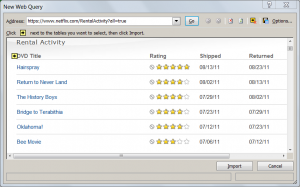When the content is transferred into the Excel spreadsheet, I saved the file (as NetflixHistory.xlsx), and closed Microsoft Excel.  The spreadsheet doesn't look like data that's ready to analyze yet (lots of extra rows and space as you can see in the example below), but that's okay.  I can fix all of that easily in SAS.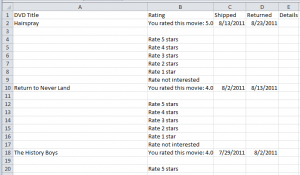With the data now in an Excel spreadsheet, I fired up SAS Enterprise Guide and selected File->Import Data. After just a few clicks through the wizard, I've got the data in a work data set.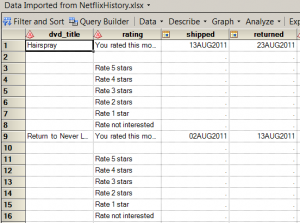## Cleaning the data and calculating the value

The data records for the account history are very simple, containing just four fields for each movie: DVD_Title, Rating (whether we liked it), Shipped (date when Netflix shipped the movie out to me), and Returned (date when Netflix received the movie back from me).  My goal for this project is to measure value, and there are no measures in this data...yet.  I also need to filter out the "garbage" rows -- those values that had some purpose in the HTML page, but don't add anything to my analysis.

I'm going to accomplish all of this within the SAS Enterprise Guide query builder, building it all into a single step.  First, I need to design a few filters to clean up the data, as shown here: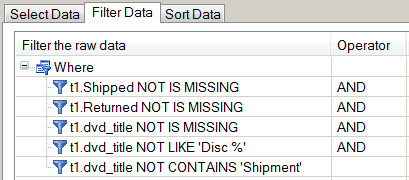The first three filters will drop all of the rows that don't contain information about a DVD title or shipment.  The last two filters will drop any records that reflect multi-disc shipments, or the occasional replacement shipment from when I reported a damaged disc.  Those are rare events, and they don't contain any information that I need to include in my analysis.

Next, I want to calculate some measures.  The most obvious measure to calculate is "How many days did we have the movie" -- the difference between the Shipped Date and Received Date.  And while that number will be interesting, by itself it doesn't convey value or cost.  I want a number that I can express in dollar terms.  To come up with that number, I will employ the tried-and-true method used by data hackers all over the world: I will Make Something Up.

In this case, I'm going to create a formula that reflects my cost for each movie.  That formula is:

(Netflix Monthly Fee / Days In a Month) * Days We Had the Movie = Cost of the Movie

Using the query builder, I calculated new columns with these values.  I assumed the fee was \$10/month (varied over time, but this constant is good enough) and that there are 30 days in a month (again, a "good enough" constant).  Here are the new columns in the query builder: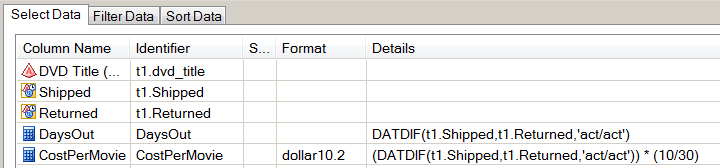After applying these filters and calculations, I finally have a result set that looks interesting to analyze: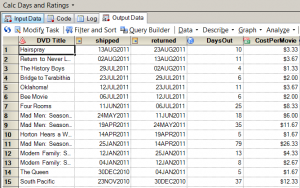By sorting this data by CostPerMovie, I can see that the "cheapest movies" were those that we had out for only 3 days, which is the fastest possible turnaround (example: receive in the mailbox on Monday, watch Monday night, mail out on Tuesday, Netflix receives on Wednesday and ships the next DVD in our queue).  By my reckoning, those DVDs cost just \$1 to watch.  The most expensive movie in my list came to \$26.33, a Mad Men DVD that sat for 79 days while we obviously had other things to do besides watch movies.

## Visualizing the results

To visualize the "Days Out" as a time series, I used the SGSCATTER procedure to generate a simple plot.  You can see that at the start of our Netflix subscription, we were enthusiastic about watching the movies immediately after we received them, and then returning them in order to release the next title from our queue.  These are where the DaysOut values are closer to zero.  But as time goes on and Life Gets Busy, there are more occurrences of "extended-period loans", with higher values for DaysOut.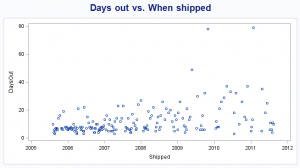Because I've calculated the cost/movie with my sophisticated model, I can plot the cost over time by using the SERIES statement in PROC SGPLOT, with this result: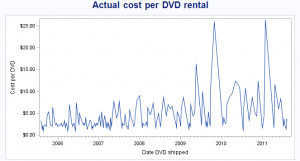This plot makes it easy to see that I've had a few "high cost" DVDs.  But it's still difficult to determine an actual trend from this, because the plot is -- and this is a technical term -- "too jumpy".  To remedy that, I used another task in SAS Enterprise Guide -- one that I probably have no business using because I don't fully understand it.  I used the Prepare Time Series Data task (found under the Tasks->Time Series menu) to accomplish two things:

• Calculate the moving average of the CostPerMovie over each 10-movie interval, in an effort to "smooth out" the variance among these values.
• Interpolate the CostPerMovie value for all dates that are covered by these data, so that on any given day I can see the "going rate" of my CostPerMovie, even if that date is not a Shipped Date or Received Date.

This magic happens behind the scenes by using PROC EXPAND, part of SAS/ETS.  And although PROC EXPAND creates some nice plots by using ODS statistical graphics, I created my own series plot again by using PROC SGPLOT: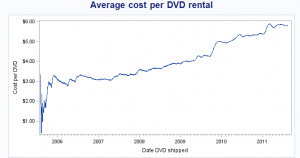This plot confirms what I already know: our movies have become more expensive over the past 6 years of my subscription.  But more importantly, it tells me by how much: from an initial cost of \$3-4, it's now up to nearly \$6 per movie -- based solely on our pattern of use.

Important note: The data I collected and analyzed covers only the DVDs we've had shipped to us.  It does not include any movies or shows that we've watched by streaming the content over the Internet.  The "instant watch" feature is an important component of the Netflix model, and we do use this quite a bit.  I know that this accounts for much of the decrease in frequency for our DVD watching.  But by changing their pricing model, Netflix basically asked the question: how much is it worth to you to continue receiving movies by mail, independent of the streaming content?

And I answered that question: it's not worth \$6 per DVD to me (as I reckon it, given my pattern of use).  Like millions of others, I've opted out of the DVD-by-mail service.  But we've kept the streaming service!  In a future post, I'll take a look at how we use the streaming content and what value we receive from it. [UPDATE: Here it is, the analysis of my streaming account.]